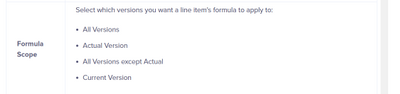# Logic Line item availble two function (Input and Formula)Hi, Everyone.

I have a question about logic Anaplan, I have a module that will create an item that can contain input and formulas, for example, for example, a period that is less than the period parameter will be blue font or input by the user and more than the period parameter will take a logic formula.

Has there been a case like this? is there a best practice?

Thank you

Tagged:

•If you are using versions, you can achieve it using Formula scope.If you are not using versions and switch over, please split the line item to two. A same line item cannot have formula and input without versions.

Thanks

Arun

•Thank you Arun, not using versions and switch over on this module. so how best bractice ?
•It is not possible to combine a formula and a user defined input in the same line item.

As described by @ArunManickam  version scope is the only instance where this is possible where you can define which version is subject to a formula and which is driven by user inputs.

The best practice here is to create multiple line items; one to capture the formula, another to contain the user input and a third to combine them based on your logic of which takes priority.

The final line item can then be used in downstream processes.

Good luck,

Chris